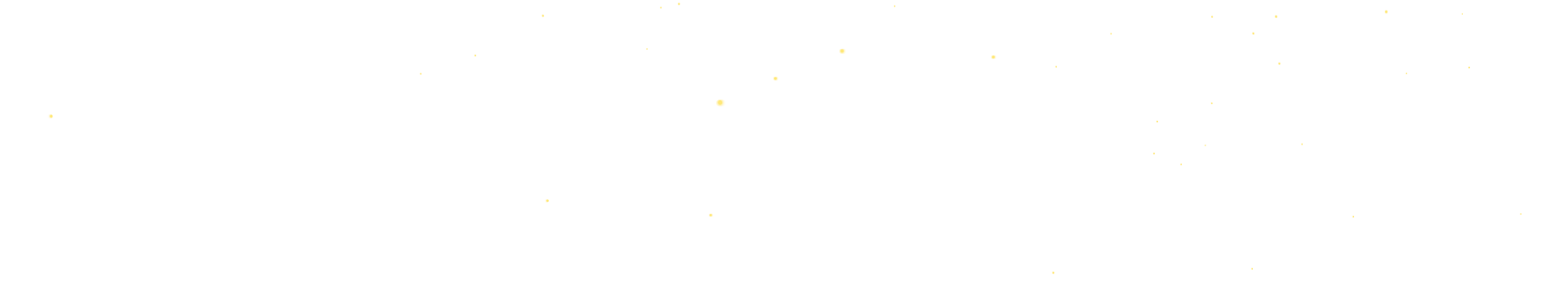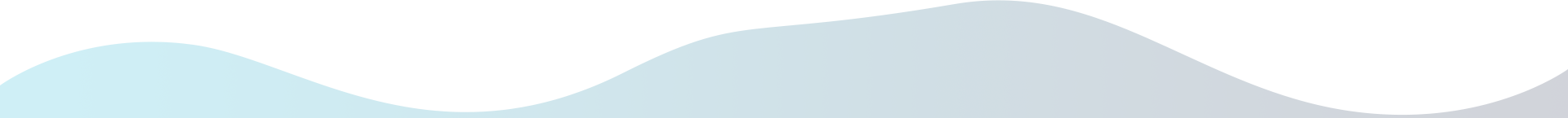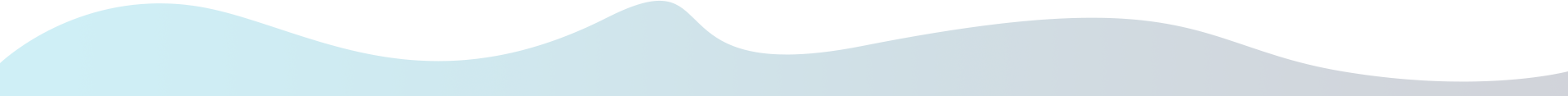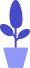# Python turtle module combat: analysis before drawing the robot cat

Estimated reading: 5 minutes 63 views

In the previous tutorial, we learned about turtle drawing. In this tutorial, we will use the knowledge introduced earlier to prepare for drawing a robot cat.

## program analysis

Let’s first take a look at the appearance of the robot cat, as shown in Figure 1.figure 1
It has a big head, round eyes, a red nose, and three beards on each side of its mouth. The head and body are separated by a red ribbon, because the robot cat in Figure 1 is sitting, so we don’t see the legs, only the round feet are exposed. In addition, the robot cat has arms and round hands. Finally, don’t forget that the Doraemon also has the iconic bell and pocket.

We can use the custom functions we learned before to define each drawing function according to the body parts of the robot cat: head (head), eyes (eyes), nose (nose), mouth (mouth), whiskers (beard), body (body), feet (feet), arms (arms), hands (hands), bell (bells) and package (pockets). The name of the function indicates which part of the body the function is responsible for drawing.

As we can see, most of these body parts consist of circles and rectangles, so in order to be able to reuse the same piece of code and avoid copying and pasting code unnecessarily, we also need to define two base functions – one is to draw The circular function drawRound(), one is the drawRect() function for drawing rectangles.

## Importing modules and setting brushes

Since we are going to use turtle drawing, we need to import the turtle module first. We’ll take the second approach of importing modules:

``````from turtle import *
``````

Using this method, you can import all the methods and variables in the turtle module, and then call the methods directly without adding the “turtle.” prefix. Now, we can write the code directly as setup(500,500) without adding a prefix and write it as turtle.setup(500,500).

Then we do some basic settings for the brush, the code is as follows.

``````#Set the window size
setup(500,500)
#setup brush
speed(10)
shape("turtle")
colormode(255)``````

Call setup(500,500) to set the canvas size to 500 pixels wide and 500 pixels high. Call speed(10) to set the brush speed to 10. Call shape(“turtle”) to set the cursor to the turtle. Call colormode(255) to set the RGB color value range from 0 to 255.

## base function

#### draw a circle

We define a drawRound() function, which we use to draw the circle. Here, two parameters are set for it, which are size representing the radius of the drawn circle and filled representing whether to fill.

• First, the pendown() function is called to indicate pen down. Then, judge whether the parameter filled is equal to True. If it is equal to True, it means to fill, then call begin_fill() function; otherwise, this function is not called, which means no filling.
• Then call setheading(180) to set the direction of movement of the turtle when it starts, that is, to make the turtle turn around. Call circle(size,360) to draw a circle with a radius of size. Then it is necessary to judge whether the parameter filled is equal to True. If it is equal to True, it means that the begin_fill() function has been called before, and the end_fill() function is called here to indicate that the filling is completed.

The code for the drawRound() function is shown below. ,

``````def drawRound(size, filled):
pendown()
if filled==True:
begin_fill()
circle(size,360)
if filled==True:
end_fill()``````

#### draw rectangle

Next, we define a drawRect() function, which we use to draw the rectangle. Here, three parameters are specified for it, which are the length representing the length of the drawn rectangle, the width representing the width of the drawn rectangle, and the filled representing whether to fill.

• First call setheading(0) to set the direction of movement of the turtle when it starts, that is, to make the turtle head to the right.
• Then call the pendown() function to indicate pen down. Determine whether the parameter filled is equal to True. If it is equal to True, it means that it needs to be filled, and the begin_fill() function is called; otherwise, the function is not called, indicating that there is no filling.
• Finally forward(length), draw an edge. Then call right(90) to rotate the cursor 90 degrees to the right. Call forward(width) to draw another edge. Call right(90) to rotate the cursor 90 degrees to the right. Call forward(length) to draw the third edge. Call right(90) to rotate the cursor 90 degrees to the right. Call forward(width) to draw the fourth edge. Then it is necessary to judge whether the parameter filled is equal to True. If it is equal, the end_fill() function is called to indicate that the filling is completed.

The code for the drawRect() function is shown below.

``````def drawRect(length,width,filled):